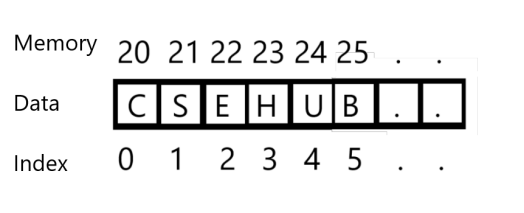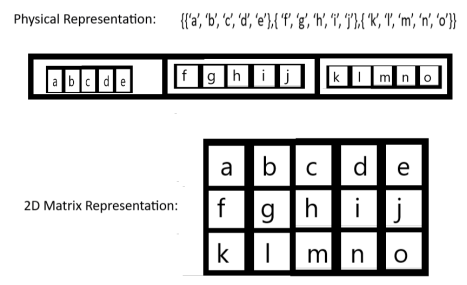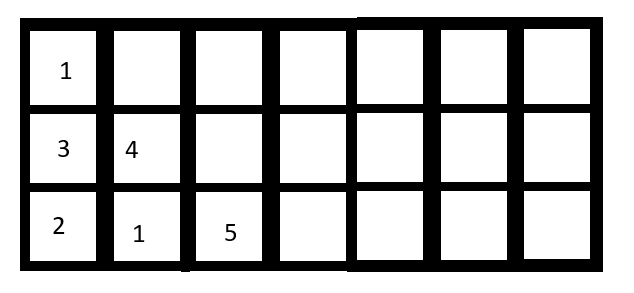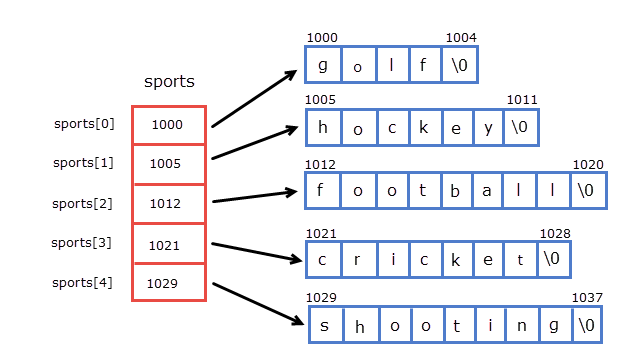Jul 05, 2018

All in one Guide to ArraysDefinition of Array

An array is a sequence of homogeneous data items stored in contiguous memory locations. For example,

• an array containing age of all students in the class,
• an array containing the name of all the citizen in the city. etc.

The definition emphasizes three points as sequential, homogeneous and contiguous.

• Sequence means each item are ordered, and their position matters.
• Homogeneous means array only consists of the same type of data elements, or all array elements have same storage size. There is a heterogeneous version of an array which we call a “list”.
• Contiguous, means one item is adjacent to other not just logically, physically as well.

As we can see the position of the elements are important so we use indices to point out each element. The first element has the index 0 next element has 1 and so on. The physical or memory address of the first element is called base address. To calculate the address of other elements we can simply use this formula:

Generally,

• the array is written as a[]
• An element of the array is written as a[i]
• The range of array items between i and j index is written as a[i:j]Different Types of indexing used in the array:

• 0 (zero-based indexing): The first element of the array is indexed by the subscript of 0. This type of indexing is most commonly used for programming.
• 1 (one-based indexing): The first element of the array is indexed by the subscript of 1.
• n (n-based indexing): The base index of an array can be freely chosen. Usually, programming languages allowing n-based indexing also allow negative index values and other scalar data types like enumerations, or characters may be used as an array index.

The array is one of the most simple data structure but it has some advantages too:

• Stores multiple elements at a time.
• Better and more convenient way of storing data.
• Multiple elements can be accessed using the same name.
• Arrays allow random access of elements. This makes accessing elements by position faster.
• Arrays have better cache locality that can make a pretty big difference in performance.
• By nesting one array into another we can create a multi-dimensional array to represent multidimensional structures.
• It can be used to implement other data structures like linked lists, stacks, queues, trees, graphs etc.

Examples –

`// An Integer array in C/C++/Javaint intArr[] = {10, 20, 30, 40, 50};// A character array in C/C++/Javachar charArr[] = {'c', 's', 'e', 'h', 'u','b'};`

From the above example,

• intArr means the 3rd element of the array which is 30.
• charArr means the 6th element of the array which is ‘b’.

Special Arrays

Multi-Dimensional Array

1. 2-D Array: 2-d Array also known as the matrix is nothing but 1-d array in another 1-d array. For example:

Let's take an array A of size 3:

`    A[] = {1,2,3}`

This array has three elements 1,2 and 3. Now, if the elements are not integer rather than they are also the array of the same size. For example:

`    A[] = {{‘a’, ‘b’, ‘c’, ‘d’, ‘e’},{ ‘f’, ‘g’, ‘h’, ‘i’, ‘j’},{ ‘k’, ‘l’, ‘m’, ‘n’, ‘o’}}`

Then we have a 2d array or matrix.2. N-D Array: Same as previous, in the N-D array each element is an (n-1)-D array.

Sparse Array:

The Sparse array is also known as the sparse matrix is a special kind of 2d array where most of the item value is empty or null. In sparse matrix ‘*’ is used to denote not null value and ‘-’ is used to denote a null value. A sparse matrix can be divided into two categories: Triangular matrix and band matrix.Pointer Array

An array of pointers is an indexed set of variables in which the variables are pointers (a reference to a location in memory). Pointers are an important tool in computer science for creating, using and destroying all types of data structures. An array of pointers is used for the same reason that all arrays are useful: it allows you to numerically index a large set of variables.Operations on an array:

Traversing:

Traversal is an operation where we need to visit each element at least once. Traversal of an array is usually done sequentially. The algorithm is pretty simple:

Input: An array A with elements, L and U as lower and upper index of array respectively.

Output: An sequence of all elements.

Algorithm:

```StartI = LWHILE I <= U DO//visiting elements	PROCESS(A[I])
I=I+1Stop```

Searching:

Searching operation is used to find an item in all of the items. The algorithm of searching an element is similar to traversal except for the process we have the comparison:

Input: An array A with elements, L and U as lower and upper index of array respectively, the search item P.

Output: The location of the first occurrence of P.

Algorithm:

```StartI = LLOC = -1WHILE I <= U DO//finding a match	IF A[I] = P THEN		LOC = I
BREAK
I=I+1
Stop```

Sorting:

Sorting operation order the array in ascending or descending manner. There are different algorithms available for sorting. We are showing the simplest one here:

Input: An array A with elements, L and U as lower and upper index of array respectively.

Output: An sequence of all elements in ascending order.

Algorithm:

```Start
I = UWHILE I >= L DO    J = L        While J < I DO    //ordering the items	IF A[J] >A[J+1] THEN		SWAP( A[J],A[J+1])
J=J+1
I=I+1
Stop```

Insertion:

Insertion of an array means adding an item to the array to some position. But not overwriting it. Insertion of one item will increase the size of the array by one. The algorithm of array insertion:

Input: An array A with elements, U as the upper index of the array, K is the item to be inserted and P is the position to be inserted.

Output: An sequence of all elements with the extra one

Algorithm:

```StartI = U+1WHILE I > P DO//shifting other elements right	A[I] = A[I-1]
I=I-1//inserting new elementsA[P] = KStop```

Deletion:

Deletion of an array means removing an item from the array. Deletion of one item will decrease the size of the array by one. The algorithm of array deletion:

Input: An array A with elements, U as the upper index of the array,  P is the position of removal.

Output: An sequence of all elements except the P positioned element.

Algorithm:

```StartI = PWHILE I < U DO//shifting other elements left
A[I] = A[I+1]
I=I+1Stop```

Merging:

Merging of arrays means compact the elements from two different arrays into a single one. The algorithm for merging:

Input: Two arrays A[L1: U1] and B[L2: U2]

Output: All elements of both arrays into a single one

Algorithm:

```Start//initialization of boundsI = 0, I2=L2, // Pouring first array
WHILE L1+I <= U1 DO
C[I] = A[L1+I]
I=I+1//Pouring second arrayWHILE L2+I-U1 <=U2 DO
C[I] = A[L2+I-U1]
I=I+1Stop```

To know more I recommend, “Classic Data Structures” by Debashish Samanta.

Assignment of Array:

Q.1: Text Based Question on Array

1. Let's assume you have an array A[-25:70], whose starting memory address is 200. Each element of the array has 3 unit allocated to them. Then find the following:

• How many elements are present in the array?
• How much memory is needed to store the array?
• What is the memory location of A?
• What are the memory location and index of the 5th element?
• What is the index of the element located at 251?

2. From whatever you have learned to find out where you can use arrays.

Q.2: Beginner Level Programming Assignments on Array:

Write programs in any programming language of all the algorithms shown here.

1. Find the sum of all elements of an array.
2. Count the total number of elements in an array.
3. Find max and min valued items in an array.
4. Find the mean of an array.

Q.3: Intermediate Level Programming Assignments on Array:

1. Modify the Traversal algorithm to reverse the array.
2. Modify the searching algorithm to find positions of all the occurrence of P.
3. Modify the searching algorithm and implement it. Assume that, all elements are sorted.
4. Modify the insertion algorithm to do a right circular shifting of an array
5. Modify the insertion algorithm for a limited size array.
6. Modify the deletion algorithm to do a left circular shifting of an array
7. Modify the deletion algorithm for a limited size array.

Q.4:

Advanced Level Programming Assignments on Array:

1. Rearrange the Array A into to B. Such that, A[i] = x becomes B[x] = I, Assume that, array A has all unique items.
2. Remove Odd numbers from an array A. e.g. A={1,2,3,4,5,6} becomes A={2,4,6}
3. Shuffle the items of an array A, and put them in C. But keep track of them using another array B.
• Write a Piece of code to Shuffle A to Produce B, C. e.g. A={1,2,3} may become C={1,3,2} and B={0,2,1}
• Write another piece of code which Produce D(clone of A) from B and C. e.g. C={1,3,2} and B={0,2,1} creates D={1,2,3}
4. Check if an array is palindrome or not. E.g. A={‘m’, ‘o’, ‘m’} or A={1,2,3,4,4,3,2,1} then it is palindrome. A={‘x’, ‘y’, ‘z’} not plaindrome.
5. Merge two array in zigzag manner. E.g. Input: A={1,2,3} B={4,5,6} Result: C={1,4,2,5,3,6}
6. Multiply two 2-d arrays or Matrix multiplication.
7. Find the number of occurances of elements in an Array. E.g. A={1,2,1,1,4,2} Result B={{1,3},{2,2},{4,0}}
8. Find the decimal value of binary array. E.g. A={1,0,1,0,1,1} Result B=43
9. Find Mean and Median of an unsorted array. E.g. A={1, 3, 4, 2, 6, 5, 8, 7} Mean = 4.5 Median=4.5
10. Delete the element whose next element is bigger. E.g. A={1,5,3} Result B={5,3}

Q.5: Expert level Programming Assignments on Array:

1. Create a student record system (roll, class, marks ) with the following features:
• Insert a new student
• Remove a Student
• Search a Student using their roll.
• Find the sort the students according to their marks.
• Group the student according to their class.
2. Merge two sorted arrays in ascending order. E.g. Input: A={1,2,7,9} B={3,5,8} Result: C={1,2,3,5,7,8,9}
3. Find Longest common subsequence between two arrays.Our Location